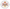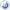# Mathematics DepartmentAuthorized school to implement the International Baccalaureate Diploma Programme (IBDP) and accredited by COGNIA for Schools (AdvancED)

### Algebra I

Algebra 1 course is intended to build a foundation for all higher math classes. This course reviews algebraic expressions, integers, and mathematical properties that will lead to working with variables and linear equations. There will be an in-depth study of graphing, polynomials, quadratic equations, data analysis and systems of equations through direct class instruction, group work, homework, student projects and technology. Students are required to use different technological tools and manipulatives to discover and explain much of the course content. The content of this course is important for students’ success on both the SAT Reasoning Test/SAT M1 and college mathematics entrance exams.  Students who complete Algebra 1 should take Geometry next. The course also emphasizes the use of the graphing calculator as a tool to interpret results as well as a method of obtaining an answer. Students in Algebra 1 are required to use a graphing calculator throughout the course.

### Geometry

The Geometry course includes an in-depth analysis of plane, solid and coordinate geometry as they relate to both abstract Mathematical concepts as well as real-world problem situations. Topics include logic and proof, parallel lines and polygons, perimeter and area analysis, volume and surface area analysis, similarity and congruence, trigonometry, analytic geometry, use relationships in circles to solve problems. Emphasis will be placed on developing critical thinking skills as they relate to logical reasoning and argument. Students will be required to use different technological tools and manipulatives to discover and explain much of the course content. The content of this course is important for students’ success on both the SAT M1/ M2 and college mathematics entrance exams.  Students who complete Algebra II should take Pre-Calculus next. Students in Geometry are required to use a graphing calculator throughout the course.

### Algebra II

The Algebra II course is designed to build on algebraic and geometric concepts.  It develops advanced algebra skills, such as systems of equations, advanced polynomials, imaginary and complex numbers, quadratics, and concepts and includes the study of trigonometric functions.  It also introduces matrices and their properties.  The content of this course are important for students’ success on both the SAT M1/ M2 and college mathematics entrance exams.  Students who complete Algebra II should take Pre-Calculus next.

The course also emphasizes the use of the graphing calculator as a tool to interpret results as well as a method of obtaining an answer. Students in Algebra II are required to use a graphing calculator throughout the course.

### Pre-calculus & Trigonometry

Pre-calculus course combines the trigonometric, geometric, and algebraic techniques needed to prepare students for the study of calculus, and strengthens students’ conceptual understanding of problems and mathematical reasoning in solving problems. Facility with these topics is especially important for students intending to study calculus, physics, and other sciences, and/or engineering in college. For this Pre-calculus course, students analyze all algebraic functions which include linear, polynomial, rational, exponential, logarithmic, trigonometric, inverse trigonometric and piecewise-defined functions. In order to prepare students for studying calculus, students will be familiarized with the properties of functions, the algebra of functions, and the graphs of functions. Students will learn the language of functions (domain and range, odd and even, periodic, symmetry, zeros, intercepts, and so on) and know the values of the trigonometric functions at the numbers on pi term. Additionally, students will learn limits and rate of change as introduction to Calculus. The course also emphasizes the use of the graphing calculator as a tool to interpret results as well as a method of obtaining an answer. Students in Pre-calculus are required to use a graphing calculator throughout the course.

### Calculus AB (AP)

AP courses in calculus consist of a full high school academic year of work, and are comparable to calculus courses in colleges and universities. It is expected that students who take an AP course in calculus will seek college credit, college placement, or both. Students are required to take the Advanced Placement Exam at the conclusion of the course.

Calculus AB is primarily concerned with developing the students’ understanding of the concepts of calculus and providing experience with its methods and applications. The course emphasizes a multi representational approach to calculus, with concepts, results, and problems being expressed graphically, numerically, analytically, and verbally. The connections among these representations also are important.  In Calculus AB course, students will learn the following main concepts: Functions, Graphs, and Limits, Derivatives and Application of Derivative, Integrals and Application of Integrals.  The course also emphasizes the use of the graphing calculator as a tool to interpret results as well as a method of obtaining an answer.  Students in Calculus AB are required to use a graphing calculator throughout the course.

### Statistics

This course prepares students to take entry level Statistics in college. Students should have a solid foundation in algebra prior to enrollment. This course introduces students to four major conceptual themes: observing and exploring data, planning a statistically valid investigation; anticipating patterns and using probability and simulations for predicting outcomes, and confirming or rejecting models through statistical inference. Technology is an integral part of the course. Graphing calculators and computers are the primary tools for data analysis.

### Accounting

This course is designed to provide students with a strong foundation in accounting principles. Students will learn what business transactions are and how accountants use a double-entry system (debits and credits) to keep track of these transactions. Students will also study the complete accounting cycle of recording transactions, preparing financial statements, and “closing the books” for small, single-owner service and merchandising businesses.

### SAT Preparation

This course is a comprehensive course that provides thorough preparation for all areas of the SAT exam. It covers content reviews to promote content mastery and teaches students the most dynamic and updated strategies and methods available. We have completely deconstructed the SAT and our students learn to think like the test makers. Students build their skills each week and increase their confidence level over the span of the course. Our SAT course consists of two weekly math classes given over one full school semester. This course requires students to work hard and exhibit effort and dedication to their studies.

Also the course includes one diagnostic SAT test and 2 mock SAT Official Full-Length Practice Tests. Students are required to take the real SAT Reasoning Exam at the end of the course.